## Biomedical Research

All submissions of the EM system will be redirected to Online Manuscript Submission System. Authors are requested to submit articles directly to Online Manuscript Submission System of respective journal.

- Biomedical Research (2016) Volume 27, Issue 1

## Clown fish queuing and switching optimization algorithm for brain tumor segmentation.

 Jobin Christ MC1*, Subramanian R2 1Department of Biomedical Engineering, Adhiyamaan College of Engineering, Hosur, India. 2Research Analyst, Student Assignment Help, New Delhi, India. Corresponding Author: Dr.MC Jobin Christ, Department of Biomedical Engineering Adhiyamaan College of Engineering Hosur India Accepted November 26, 2015
Visit for more related articles at Biomedical Research

### Abstract

Segmentation of an image is the separation or division of the image into diverse regions of similar feature. In medical field, magnetic resonance image (MRI) is used to discriminate pathological tissues from normal tissues, particularly for brain tumors. Many methods are proposed and already in use for the tumor segmentation. In this paper, we have proposed a new sophisticated algorithm for the segmentation of the brain tumor. The image is segmented using swarm intelligence based approach by characterizing the ecological behaviour of clown fish. The algorithm is based on the queuing characteristics of the clown fish. In this paper a step by step methodology for the automatic MRI brain tumor segmentation is presented. Initially acquired MR brain images are divided into two approximately symmetric halves. Next skull is detected. Next an ellipse is fitted to the skull boundary, from which the line of symmetry is extracted. Final step is the tumor detection. The results of the proposed method are compared with well known other algorithms like Fuzzy C-Means clustering, self-organizing map and particle swarm optimization. The results show that the proposed algorithm is a promising method to segment the brain tumors accurately. Using Clown fish queuing and optimization algorithm, we achieved 100% sensitivity and 98% accuracy.

### Keywords

Brain Tumor; Segmentation; Optimization algorithm; Medical Image; MRI

### Introduction

A brain tumor is an intracranial solid neoplasm, a tumor (defined as an abnormal growth of cells) within the brain or the central spinal canal. Some tumors are brain cancers. Brain tumors may also spread from cancers primarily located in other organs (metastatic tumors) . Brain tumors are the second leading cause of cancer-related deaths in children under age 20. They are the second leading cause of cancer-related deaths in males ages 20-39. Brain tumor is the fifth leading cause of cancer-related deaths in females’ ages in between 20 and 39 . So early detection of brain tumor is the only way to minimize the death rate.
Image segmentation algorithms are normally classified into two types, supervised and unsupervised. Unsupervised algorithms are fully automatic methods and separate the regions into feature space with high density. The various unsupervised algorithms are feature space based techniques, histogram thresholding, clustering techniques (K-means algorithm, C-means algorithm, E-means algorithm, etc.), region based techniques (split and merge techniques, region growing techniques), artificial neural network based techniques, fuzzy techniques, edge detection techniques, etc.
The K-means algorithm is an iterative technique that is used to partition an image into K clusters. K can be selected manually, randomly, or by a heuristic as explained by J. Selvakumar et al. . This algorithm is guaranteed to converge, but it may not return the optimal solution. Like the K-Means algorithm, the Fuzzy C-Means (FCM) aims to minimize an objective function. The FCM algorithm is better than the K-mean algorithm, since in K-mean algorithm, feature vectors of the data’s set can be partitioned into hard clusters, and the feature vector can exactly be a member of one cluster only as depicted by J. Selvakumar et al. .
Particle swarm optimization (PSO) is a stochastic search method that was developed in 1995 based on the sociological behaviour of bird flocking. Now, the PSO technique has been used to solve the problem of thresholding based segmentation. Zahara et al. combined a hybrid Nelder–Mead simplex search method and the PSO technique to solve the objective functions of Gaussian curve fitting and the Otsu's method. This combination is applied to image thresholding with multi-modal histograms . In a multilevel threshold selection based on PSO was proposed. The PSO technique was used to find near-optimal thresholds by minimizing the cross entropy between the original image and its thresholded version. Maitra et al., proposed a new thresholding algorithm for histogram-based image segmentation using a hybrid cooperative-comprehensive learning based on the PSO algorithm .
Reddick et al., developed a pixel based two stage approach where a self organizing map (SOM) was trained to segment multispectral MR images into white matter, gray matter, etc., by a feed forward artificial neural network . Vijayakumar et al., proposed a method based on SOM to segment tumor, necrosis, cysts, edema, and normal tissue in MRI . Iftekharuddin et al. presented a method where the effectiveness of two novel fractal and fractal wavelet features was exploited to segment and classify tumor and non tumor regions along with intensity values in multimodal MR images . Murugavalli et al., implemented a hybrid technique combining the advantages of a hierarchical self-organizing map (HSOM) and Fuzzy C-Means clustering (FCM) to detect various tissues like white matter, gray matter, cerebrospinal fluid and tumor in MR images .

### Materials and Methods

In this paper, a step by step methodology for the automatic MRI brain tumor segmentation is presented. Initially acquired MR brain images are divided into two approximately symmetric halves. Next skull is detected. Next an ellipse is fitted to the skull boundary, from which the line of symmetry is extracted. Final step is the tumor detection using proposed algorithm. In this paper, we have developed a new sophisticated algorithm called clown fish queuing and switching optimization algorithm (CFQSOA) for the segmentation of the brain tumor image. The algorithm is based on the queuing characteristics of the clown fish. The clown fish is a type of fish, which attach themselves and survive symbiotically with the sea anemones. The clown fish ecological behavior is to acquire the social rank in the dominant position in the shorter queues in sea anemone. The female clown fish are mostly dominant and large which are denoted as alpha. The male clown fish are small and denoted as beta. There are some sub ordinates and immature fishes represented as gamma. If female fish removed or died, the male fishes acquire their places with shorter queues. Then the male fish become dominant female fishes and the new sub ordinate will become new male to acquire their social ranks in the shorter queues. The basic behaviors of CFQSO are Prey, Swarm, Follow, and Switch where they search for dominant position searching, and these behaviors are based on the artificial fish Swarm intelligence as depicted by Zhehuang Huang et al. . Figure 1 shows the flow diagram of the proposed algorithm.
The present position of dominant fish “i” is declared as Xi and the position of fish “j” is declared as Xj. The distance is declared as Euclidean distance which is given as follows.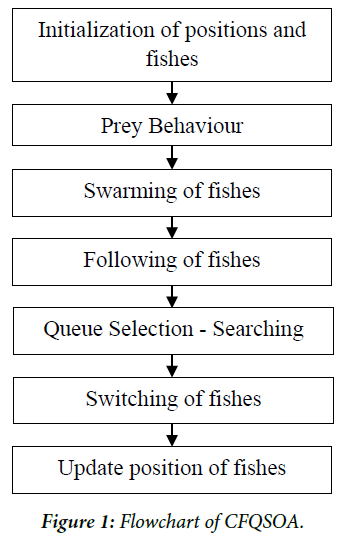Visual and δ represent the visual distance and crowd factor of the Artificial Fish (AF), the visual distance is the distance at which the fish locates, and the crowd factor is termed as the degree of freedom at which the fish moves. It is represented as δ.
nf is the number of its fellow within the Visual. Mstep is the maximum step of the AF moving; Step is a random positive number within Mstep;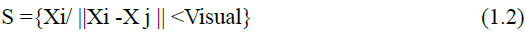is the set of fish j exploring area at the present position. Y=f(X) is the fitness function for reaching the dominant position.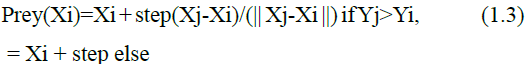Swarm(Xi)=Xi + step(Xc-Xi)/(|| Xc-Xi||) if Yc/fish_no> crowd factor
= prey(Xi)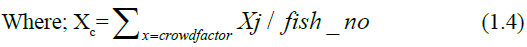else,
Follow(Xi) = Xi + step(Xmax-Xi)/(||Xmax-Xi||)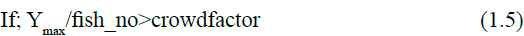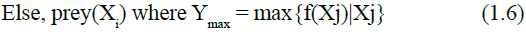The total number of fishes assigned in the project is about 100. In image processing, the algorithm is implemented. The fish moves when the algorithm is operated into the image thus the real time simulation of the movement of the fishes in the form of cells can be viewed in the image. The simulation occurs till the search optimization is completed in acquiring the minimum threshold. As the threshold is the minimum function, the fish will acquire the process of the queuing and switching till it reaches its optimized state. Finally when the optimized state is acquired, in other words the alpha is Zero (female fish dies or moved), the male acquires the dominant position where the beta will be One. Thus the tumor segmented part will be white in color before switching (i.e., alpha=1, beta=0). While in the condition of after switching, the tumor segmented part is acquired by the male fish which shows the tumor as black in color. (i.e., beta=0, alpha=1). Thus the process is reciprocated after switching.
The algorithm of the CFQSOA is given below,
ii. Initialize the no. of fishes.
iii. Crowd factor is a term that influences the speed of the movement of the fishes.
iv. Linearly map the image I and J, where image I is the input brain tumor image and image J is the binary image of I.
v. Declare the direction of the fish to move in four directions.
vi. Use the formula for step, prey, swarm and follow.
vii. Update the values of step, prey, swarm and follow.
viii. Find the gradient of the image.
ix. Find the threshold of the image.
x. Find the step of the fishes and update the position of fishes in image.

### Results and Discussion

In this paper, brain tumors are segmented by using the CFQSOA and characterizing the ecological behavior of the clown fish. The results are produced by MATLAB R2010a version in Windows 7 OS, in the Pentium processor system. The proposed system’s results are shown in Figure 2. The proposed system is tested with 100 brain tumor images which have 95 images with tumor and 5 without tumor. The images considered are 1024 × 1024 pixel images in the .jpeg file extension. The tumor images used in the paper for the segmentation are the tumors such as glioma and meningioma.
The statistical analysis is performed with the 100 images in which the 95 images are with tumor and 5 images are normal. Then the testing is performed to segment the tumor for positive and negative analysis. The proposed method is used for further analysis for prediction of the sensitivity, specificity, positive prediction value and negative prediction value.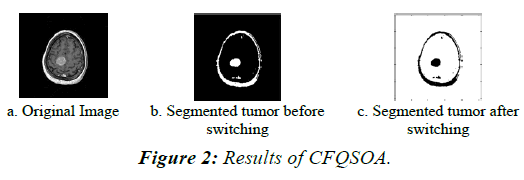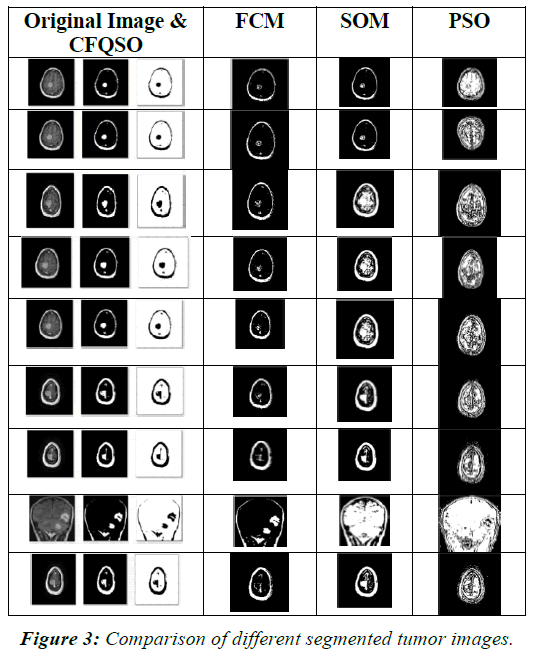Specificity=TN/(TN+FP)
Accuracy=TP + TN/(TP+FP+TN+FN)
Where, TP=True Positive, TN=True Negative, FP=False Positive, FN=False Negative
Our algorithm segments the tumor more accurately than the FCM, SOM and PSO methods. The output images are shown in the Figure 3. Comparison of execution times is given in Figure 4. The executive time seems to be very less in our proposed system such that it takes a time period of 12 seconds. Parameters used in our methodology, Mean of parameters, Comparative analysis of the execution time for segmentation, Positive and negative rates for different methods, Comparison of parameters and Accuracy analysis are listed in Table 1 to Table 6 respectively.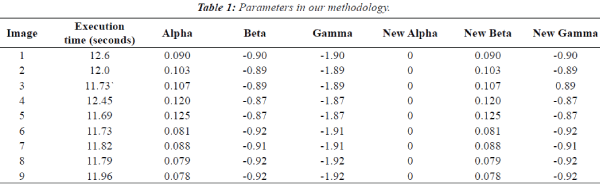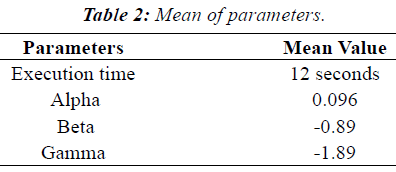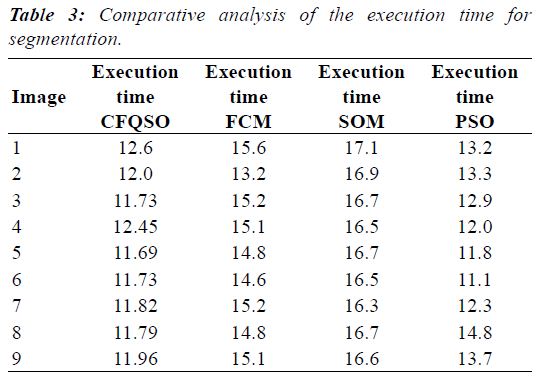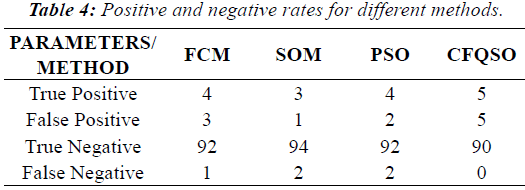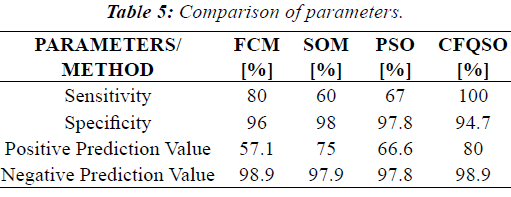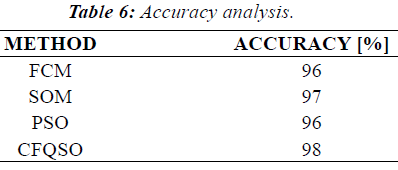### Conclusion

In this paper, we have proposed a new sophisticated algorithm named Clown Fish Queuing and Switching Optimization Algorithm (CFQSOA) for the segmentation of the brain tumor. The image is segmented using swarm intelligence based approach by characterizing the ecological behaviour of clown fish. The results of the proposed method are compared with well known other optimization algorithms. The results show that the proposed algorithm is a promising method to segment the brain tumours accurately. Using Clown fish queuing and optimization algorithm, we achieved 100% sensitivity and 98% accuracy. In future this algorithm may be extended with brain tumor classification based on the characteristics of area and volume, features, using neural networks, fuzzy logic or some hybrid techniques by extracting the features for training and testing.